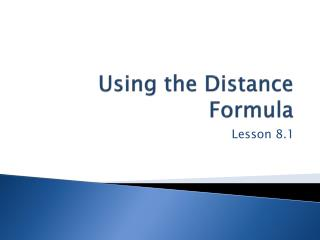Download PresentationUsing the Distance Formula

# Using the Distance Formula - PowerPoint PPT PresentationDownload Presentation## Using the Distance Formula

- - - - - - - - - - - - - - - - - - - - - - - - - - - E N D - - - - - - - - - - - - - - - - - - - - - - - - - - -
##### Presentation Transcript

1. Using the Distance Formula Lesson 8.1

2. Imagine a race in which you carry an empty bucket from the starting line to the edge of a pool, fill the bucket with water, and then carry the bucket to the finish line. Luck, physical fitness, common sense, a calm attitude, and a little mathematics will make a difference in how you perform. Your performance depends on your speed and on the distance you travel. As you’ll see in the investigation, mathematics can help you find the shortest path.

3. Bucket Race • The starting line of a bucket race is 5 m from one end of a pool, the pool is 20 m long, and the finish line is 7 m from the opposite end of the pool, as shown. In this investigation you will find the shortest path from point A to a point C on the edge of the pool to point B. That is, you will find the value of x, the distance in meters from the end of the pool to point C, such that AC CB is the shortest path possible.

4. Step 1 Make a scale drawing of the situation on graph paper. • Step 2 Plot several different locations for point C. For each, measure the distance x and find the total length AC CB. Record your data. • Step 3 What is the best location for C such that the length AC BC is minimized? What is the distance traveled? Is there more than one best location? Describe at least two different methods for finding the best location for C. • Step 4 Make a scale drawing of your solution.

5. Imagine that the amount of water you empty out at point B is an important factor in winning the race. This means you must move carefully so as not to spill water, and you’ll be able to move faster with the empty bucket than you can with the bucket full of water. Assume that you can carry an empty bucket at a rate of 1.2 m/s and that you can carry a full bucket, without spilling, at a rate of 0.4 m/s.

6. Step 5 Go back to the data collected in Step 2 and find the time needed for each x-value. • Step 6 Now find the best location for point C so that you minimize the time from point A to the pool edge, then to point B. What is your minimum time? What is the distance traveled? How does this compare with your answer in Step 3? Describe your solution process.

7. Example A • An injured worker must be rushed from an oil rig 15 mi offshore to a hospital in the nearest town 98 mi down the coast from the oil rig. • Let x represent the distance in miles from the point on the shore closest to the oil rig and another point, C, on the shore. How far does the injured worker travel, in terms of x, if a boat takes him to C and then an ambulance takes him to the hospital? • Assume the boat travels at an average rate of 23 mi/h and the ambulance travels at an average rate of 70 mi/h. What value of x makes the trip 3 h?

8. One way to find the value of x that gives a trip of 3 h is to graph the total time equation and y =3, and use the graph to approximate the intersection. The graphs intersect when x is approximately 51.6. For the trip to be 3 h, the boat and the ambulance should meet at the point on the shore 51.6 mi from the point closest to the oil rig.

9. An equation that describes a set of points that meet a certain condition is called a locus. For example, the locus of points that are 3 units from the point (0, 0) is the circle with equation x2 +y2 = 9. The locus of points midway between (4, 0) and (4, 0) is the line x 0, the y-axis. In this chapter you will explore equations describing a variety of different loci (the plural of locus).

10. Example B • Find the equation of the locus of points that are equidistant from the points (1, 3) and (5, 6).

11. First make a sketch of the situation. Plot the two given points and then estimate the location of a few points that are the same distance from the given points. Do they appear to be in any sort of pattern? • In this case they appear to lie on a line. Therefore, you should expect to find the equation of a line as your final answer. Let d1 represent the distance between (1, 3) and any point, (x, y), on the locus. By the distance formula,

12. Let d2 represent the distance between (5, 6) and the same point on the locus, so • The locus of points contains all points whose coordinates satisfy the equation d1=d2, or

13. As you predicted, the locus is a line. It has equation, How could you show that this locus is the perpendicular bisector of the segment joining the two points?